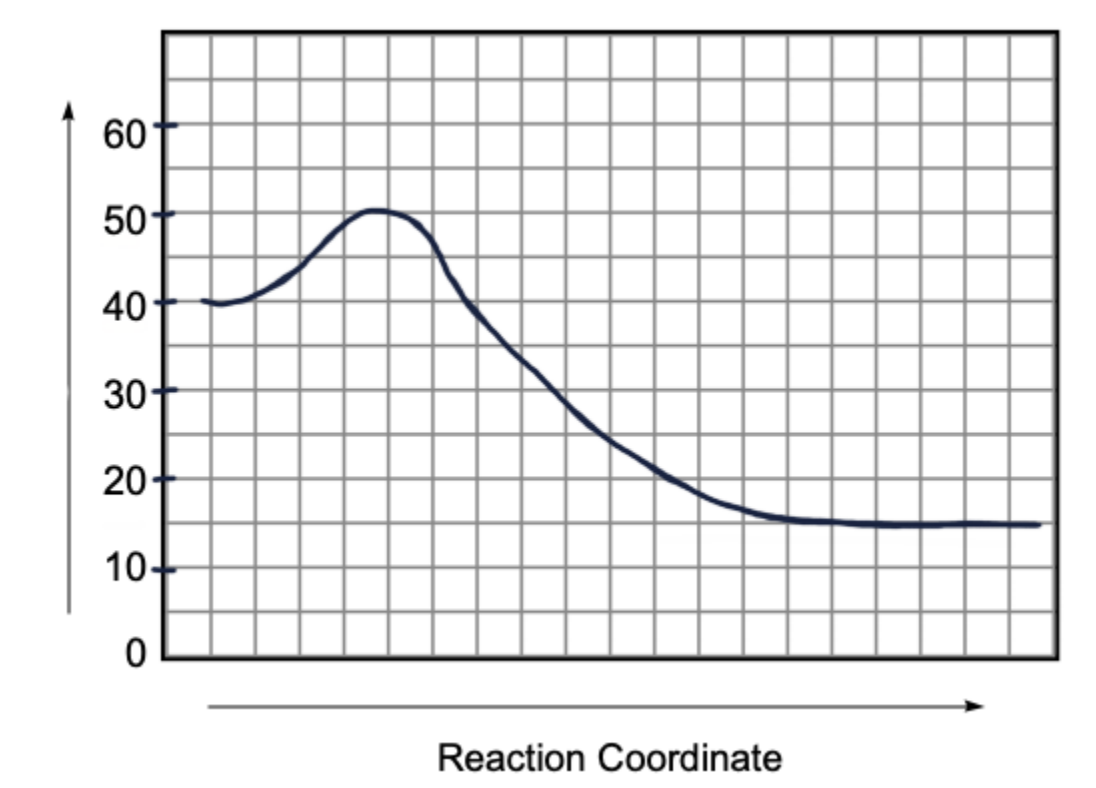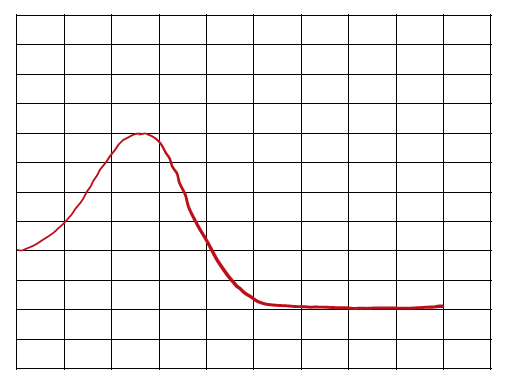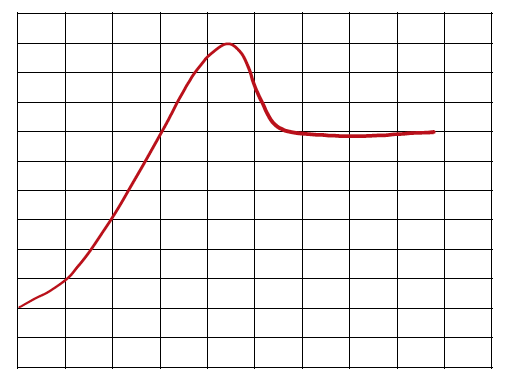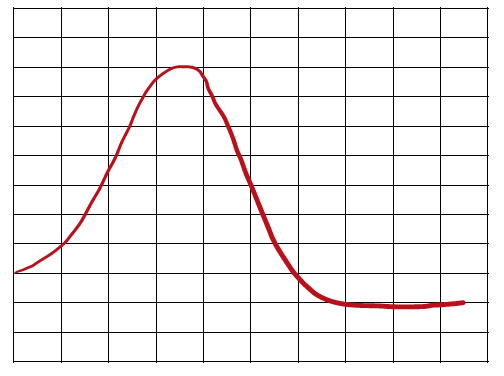Clutch Prep is now a part of Pearson
Ch.7 Energy, Rate and EquilibriumWorksheetSee all chapters

# Energy Diagrams

See all sections
Sections
Nature of Energy
First Law of Thermodynamics
Endothermic & Exothermic Reactions
Bond Energy
Thermochemical Equations
Heat Capacity
Thermal Equilibrium (Simplified)
Hess's Law
Rate of Reaction
Energy Diagrams
Chemical Equilibrium
The Equilibrium Constant
Le Chatelier's Principle
Solubility Product Constant (Ksp)
Spontaneous Reaction
Entropy (Simplified)
Gibbs Free Energy (Simplified)

An Energy Diagram is a curved plot on a graph that Illustrates the energies of reactants, products and transition state as a reaction occurs.

###### Energy Diagram

Concept #1: Energy Diagram

Example #1: What is the energy value for the product within the following energy diagram?

Concept #2: Speed of Reactions

Example #2: Which reaction will occur in the shortest amount of time?

a) Reaction A (Ea = 143 kJ)

b) Reaction B (Ea = 80 kJ)

c) Reaction C (Ea = 215 kJ)

Concept #3: Stability

Practice: Which of the following statements is true regarding the energy diagram provided?i. The reaction is endothermic.
ii. The activation energy is +10 kJ.
iii. The reaction releases energy.
iv. The enthalpy of the reaction is –25 kJ.

Practice: Which of the following reactions proceeds the slowest?Reaction 1                                             Reaction 2                                       Reaction 3